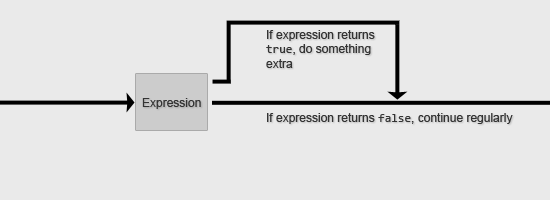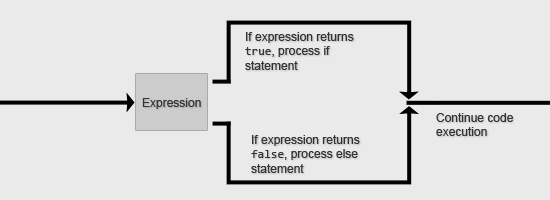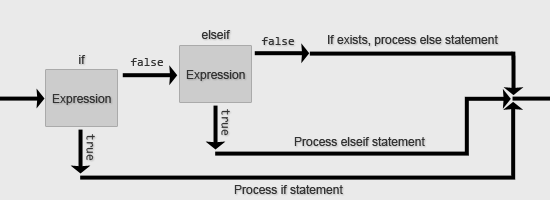# Learning PHP: Working with Conditional StatementsIn this PHP guide, we will take a look at conditional statements in PHP. Conditional statements — also known as conditional statements, conditional expressions or conditional constructs — are a group of programming features that can be found in every programming language. An example of a conditional statement is the if statement.

There are several conditional statements in PHP, and it is up to you as a programmer to determine which approach to take in a given situation.

To get the most out of this guide, you should have already read the following:

### Introduction

A conditional statement, in essence, helps a program decide which route to take based on how the condition is evaluated. Conditional statements are evaluated as either being true or false.

The simplest conditional statement is an if statement. For example, if it is morning, the sun is rising.### The Basics of Building Conditions

There are two basic concepts that will help you build conditions. The first concept is that a condition always returns true or false. The second concept is that as long as something has (or returns) a value — and almost everything in PHP does — it can be used in a condition.

Let’s start with a basic example which checks whether `\$player_name` equals Freddy. To determine how our condition is evaluated, we will use `var_dump()`, which we used in the PHP variables guide to view the values inside variables and arrays.

```<?php
\$player_name = 'Nathan';
var_dump(\$player_name == 'Freddy');
?>```

The condition can be translated to English as: Does the \$player_name variable equal Freddy?. In this case, `var_dump()` will print `false` because `\$player_name` is equal to Nathan.

### Comparison Operators

The `==` between `\$player_name == 'Freddy'` is called a comparison operator. More specifically, it is the Equal comparison operator. A comparison operator compares between two values. It is the bread and butter of conditional statements because it is the basis in which you build your conditions upon.

All the comparison operators available in PHP can be found in the table below. This table is adapted from the official PHP documentation of Comparison Operators. Please take a moment to go over this table before going any further.

Example Name Result
\$a == \$b Equal `true` if `\$a` is equal to `\$b`.
\$a === \$b Identical `true` if `\$a` is equal to `\$b`, and they are of the same variable type.
\$a != \$b Not equal `true` if `\$a` is not equal to `\$b`.
\$a <> \$b Not equal `true` if `\$a` is not equal to `\$b`.
\$a !== \$b Not identical `true` if `\$a` is not equal to `\$b`, or if they are not of the same variable type.
\$a < \$b Less than `true` if `\$a` is less than `\$b`.
\$a > \$b Greater than `true` if `\$a` is greater than `\$b`.
\$a <= \$b Less than or equal to `true` if `\$a` is less than or equal to `\$b`.
\$a >= \$b Greater than or equal to `true` if `\$a` is greater than or equal to `\$b`.

### Combining Multiple Comparisons

Let’s say that you have an input field where a user needs to enter a value between 0 and 11 (i.e. 1 – 10 are valid inputs). You put their input into a variable called `\$rating`. The user enters -3 so `\$rating` is equal to -3.

We have two comparisons that must be true for the condition (otherwise known as a comparison expression in PHP) to be true. First, it must be greater than 0. Secondly, it must be less than 11.

Here’s how we can write our comparison expression:

```<?php
\$rating = -3;
var_dump(\$rating > 0 && \$rating < 11); // Outputs false
?>
```

The `&&` between the two comparisons is called a logical operator. A logical operator allows us to make multiple comparisons within a comparison expression. The logical operator above is called the And logical operator. There are four types of logical operators: And, Or, Xor, and Not.

In the above example, our comparison expression in English translates to: Is \$rating greater than zero and is \$rating less than eleven?

Since `\$rating` is -3, though it evaluates true for the second comparison (`\$rating < 11`), it evaluates false for the first one (`\$rating > 0`) and thus the entire comparison expression is false because both comparisons were not true.

What if you want the condition to evaluate true if just one of the comparisons is true? We can use the Or logical operator, which is represented by ||.

Let’s say that we have an input field that allows users to enter a location (such as a city in Europe). We want the condition to be true if they type Berlin or Amsterdam. Let us say that they entered Berlin and you placed that input value into a variable called `\$location`.

Here is how we would write the conditional expression stated above:

```<?php
\$location = 'Berlin';
var_dump(\$location == 'Berlin' || \$location == 'Amsterdam'); // true
?>
```

The conditional expression above is true because `\$location` is equal to Berlin. Unlike the And logical operator, the Or logical operator will evaluate a condition as true even if only one of the comparisons is true. If the user enters Chicago, then the condition above will be false because Chicago is neither Berlin nor Amsterdam.

Below is a table listing all four logical operators in PHP. `&&` and `||` can also be written as `and` or `or`. It is, however, best practice to just use `&&` and `||` for standardization. This table is adapted from the official PHP documentation of Logical Operators.

Example Name Result
\$a and \$b And `true` if both `\$a` and `\$b` are true.
\$a or \$b Or `true` if either `\$a` or `\$b` is true.
\$a xor \$b Xor `true` if either `\$a` or `\$b` is true, but not both.
!\$a Not `true` if `\$a` is not true
\$a && \$b And `true` if both `\$a` and `\$b` are true.
\$a || \$b Or `true` if either `\$a` or `\$b` is true.

### Conditional Statements

The conditional statements in PHP are if, else, elseif, and switch. Let’s go over each one.

#### The if Statement

The if statement is a fundamental building block of many programming and scripting languages – PHP is no exception. With the if statement, a developer is able to create simple control structures.An if statement can be read as: if the condition is true, perform this stuff, otherwise ignore this stuff.

Here is an example that prints out the string assigned to `\$message`. If `\$name` is equal to Freddy, this script will print Hi Freddy! If `\$name` is not equal to Freddy, it will simply print Nice name!

```<?php
\$name = 'Nathan';
\$message = 'Nice name!';

if (\$name == 'Freddy')
{
\$message = 'Hi Freddy!';
}

echo \$message;
?>```

As you can see above, we can perform different things based on the result of the comparison expression. In this case, if `\$name` is equal to Freddy, then we alter the value of `\$message`; otherwise we go on and keep the value of `\$message` the same.

The code inside the curly brackets (`{...}`) will be processed when the expression used in the if statement evaluates to true.

You can choose not to include curly brackets if you only have one expression to execute (e.g. only one line of code, denoted by the `;` at the end of it). However, using curly brackets is commonly acknowledged as being more readable.

Without curly brackets, we can rewrite the example above as follows:

```<?php
\$name = 'Nathan';
\$message = 'Nice name!';

if (\$name == 'Freddy')
\$message = 'Hi Freddy!';

echo \$message;
?>```

Last but not least: if statements can be nested within other if statements. Making use of nested if statements will provide you with ultimate flexibility for conditional execution of the various code paths of your script.

In the following nested if statement example, we use the `date()` function to get the time of day (in 24-hour format). If `\$name` is equal to Freddy, the `\$message` value will be changed to Good afternoon or evening Freddy!. Afterwards, it also evaluates the if statement nested inside it. If `\$time` is less than 12 (i.e. it is between 1:00AM-12:00PM), then `\$message` will be changed again to say Good morning Freddy!.

If `\$name` is not Freddy, nothing inside the first if statement will execute, which also means the nested if statement will not be evaluated.

```<?php
\$time = date(H); // Get time in 24-hour format

\$message = 'Nice name!';

if (\$name == 'Freddy')
{
\$message = 'Good afternoon or evening Freddy!';
if (\$time < 12)
{
\$message = 'Good morning Freddy!';
}
}

echo \$message;
?>```

#### The else Statement

The else statement extends the if statement. Because the else statement cannot hold a comparison expression, you can only make use of it in combination with an if statement. The else statement executes code if the if statement’s comparison expression evaluates to false.Here is an example that will behave differently depending on the value of `\$rating`:

```<?php
\$rating = -3;

if (\$rating > 0 && \$rating < 11)
{
echo 'This rating is not valid.';
}
else
{
echo 'Thanks for rating!';
}
?>```

Self-quiz #1: What does echo output in the code block above? (See answer below.)

Just like if statements, else statements can be nested within other if statements:

```<?php
\$rated = false;
\$rating = 8;

if (\$rating > 0 && \$rating < 11)
{
echo 'This rating is not valid, please try again.';
}
else
{
// User has already rated in the past
if (\$rated)
{
echo 'You are not allowed to rate twice.';
}
else
{
// Store rating
echo 'Thanks for rating!';
\$rated = true;
}
}
?>```

#### Ternary Operator

The ternary operator in PHP is a conditional operator that follows the if/else control structure. It can be used as shorthand for when you are writing an if/else statement that assigns a value to a variable. The structure of the ternary operator is as follows:

`\$foo = ([conditional expression]) : [value if true] ? [value if false];`

Here is an if/else control structure that assigns `\$b` a string value depending on the value of `\$a` — we will translate the following code to the ternary operator afterwards.

```<?php
if (\$a < 0)
{
\$b = 'Apple';
}
else
{
\$b = 'Banana';
}
?>```

The above control structure can be rewritten using the ternary operator as such:

```<?php
\$b = (\$a < 0) : 'Apple' ? 'Banana';
?>```

#### The elseif Statement

The elseif statement extends the else statement so that it can have a comparison expression. If the elseif condition is true, it will (just like the if statement) execute the statements within its curly brackets.You may add as many elseif statements to an if statement as you like. You could also have an else statement at the end as a catchall when the if statement and elseif statements all evaluate false. If there is no else statement at the end of the control structure, PHP just continues.

The following example is a simplified control structure for accessing a web page. Let us say that you have asked for the username and password of the site visitor to see if he or she can access a web page. Let us also say that you stored the username and password in the variables `\$username` and `\$password`. However, we only have three username/password combinations that can access this page. Everyone else will be denied access (i.e. `\$access = false`).

```<?php
\$access = false;

{
\$access = true;
}
{
\$access = true;
}
{
\$access = true;
}
else
{
\$access = false;
}
?>```

Self-quiz #2: What is the value of `\$access` after the script above runs? (See answer below.)

Note that in PHP, you can also write else if (two words) instead of elseif. The behavior would be identical to elseif. The syntactic meaning is slightly different but you can assume that both would result in exactly the same behavior. Best practice suggests using elseif over else if.

#### The switch Statement

The switch statement is used to check a variable (or comparison expression) against many different values.Switch statements can usually be rewritten as multiple if statements.

Here is an example of a switch statement. The switch evaluates the value of `\$username`, and then assigns `\$rank` depending on its value.

```<?php
\$rank = 0; // The key which associates with the \$ranks array

{
case 'Nathan':
\$rank = 2;
break;

case 'Tim':
\$rank = 1;
break;

case 'Freddy':
\$rank = 1;
break;
}

echo 'Hello ' . \$username . '! Your rank is ' . \$ranks[\$rank] . '.';
?>```

Self-quiz #3: What is outputted by the echo statement? (See answer below.)

Switch statements can have multiple cases assigned to the same code block. In the above example, we see that `case 'Tim'` and `case 'Freddy'` do the same thing (i.e. it assigns `\$rank = 1`), so we can rewrite the above as:

```<?php
\$rank = 0;

{
case 'Nathan':
\$rank = 2;
break;

case 'Tim':
case 'Freddy';
\$rank = 1;
break;
}

echo 'Hello ' . \$username . '! Your rank is ' . \$ranks[\$rank] . '.';
?>```

You can use default as the catchall case, similar to how you would use an else at the end of a series of if statements. If none of the cases match the switch conditional expression, then default will be executed.

```<?php
\$rank = 0;

{
case 'Nathan':
\$rank = 2;
break;

case 'Tim':
case 'Freddy':
\$rank = 1;
break;

default:
\$rank = 0;
break;
}

echo 'Hello ' . \$username . '! Your rank is ' . \$ranks[\$rank] . '.';
?>```

### Conclusion

This guide covered the fundamentals of conditional statements in PHP. We discussed comparison operators, logical operators and the if, elseif, and switch conditional statements. We also discussed the ternary operator, which follows the if/else control structure.

1. This rating is not valid.
2. The value of `\$access` is false.
3. Hello Freddy! Your rank is member.

### Related ContentElias Zerrouq is a young web developer located in the Netherlands. He specializes in back-end development (PHP and MySQL) and classifies himself as a non-designing web developer (front-end development is okay though). Contact information can be found through his personal website.